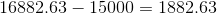# PSAT Math : How to find compound interest

## Example Questions

### Example Question #1 : Pattern Behaviors In Exponents

On January 15, 2015, Philip deposited $10,000 in a certificate of deposit that returned interest at an annual rate of 8.125%, compounded monthly. How much will his certificate of deposit be worth on January 15, 2020? Possible Answers: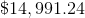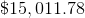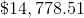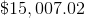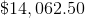Correct answer:Explanation: The formula for compound interest is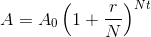where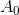is the initial investment,is the interest rate expressed as the decimal equivalent,is the number of periods per year the interest is compounded,is the number of years, andis the final value of the investment. Set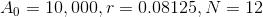(monthly = 12 periods), and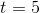, and evaluate: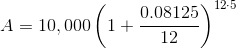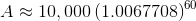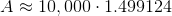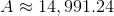The CD will be worth$14,991.24.

### Example Question #2 : Pattern Behaviors In Exponents

Money is deposited in corporate bonds which yield 6.735% annual interest compounded monthly, and which mature after ten years. Which of the following responses comes closest to the percent by which the value of bonds increases?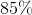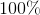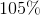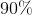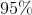Explanation:

The formula for compound interest iswhereis the initial investment,is the interest rate expressed as the decimal equivalent,is the number of periods per year the interest is compounded,is the number of years, andis the final value of the investment.

In the given scenario,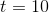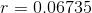, and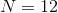(monthly); substitute: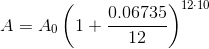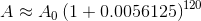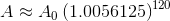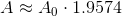This meas that the final value of the bonds is about 1.96 times their initial value, or, equivalently, 96% greater than their initial value. Of the given responses, 95% comes closest.

### Example Question #3 : Pattern Behaviors In Exponents

Donna wants to deposit money into a certificate of deposit so that in exactly ten years, her investment will be worth $100,000. The interest rate of the CD is 7.885%, compounded monthly. What should Donna's initial investment be, at minimum? Possible Answers: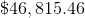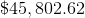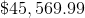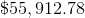More information is needed to answer the question. Correct answer:Explanation: The formula for compound interest iswhereis the initial investment,is the interest rate expressed as the decimal equivalent,is the number of periods per year the interest is compounded,is the number of years, andis the final value of the investment. Set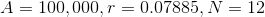(monthly = 12 periods), and, and evaluate: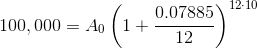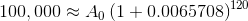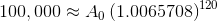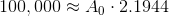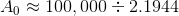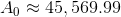The correct response is$45,569.99.

### Example Question #4 : Pattern Behaviors In Exponents

Tom invests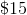,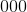in a savings account with an annual interest rate of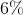. If his investment is compounded semiannually, how much interest does he earn afteryears?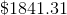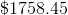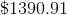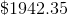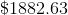Explanation:

In order to find the interest earned, used the compound interest formula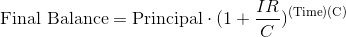whererepresents the number of times the account is compounded each year, and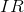represents the interest rate expressed as a decimal.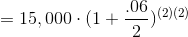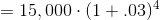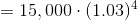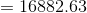The account is worth $16882.63 after two years. Therefore Tom earns$1882.63 in interest.